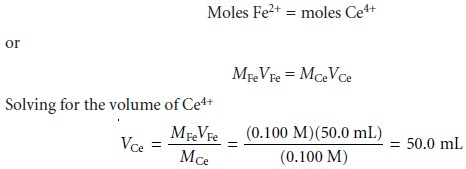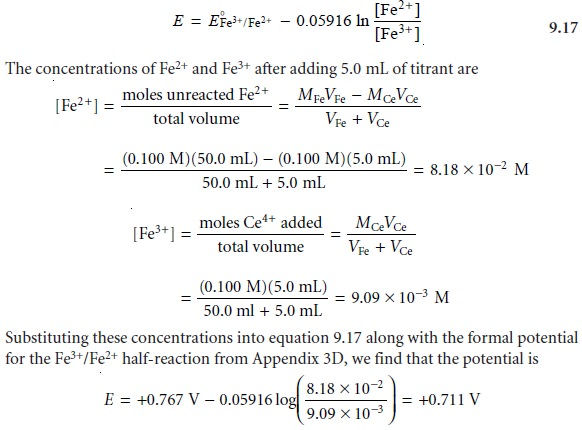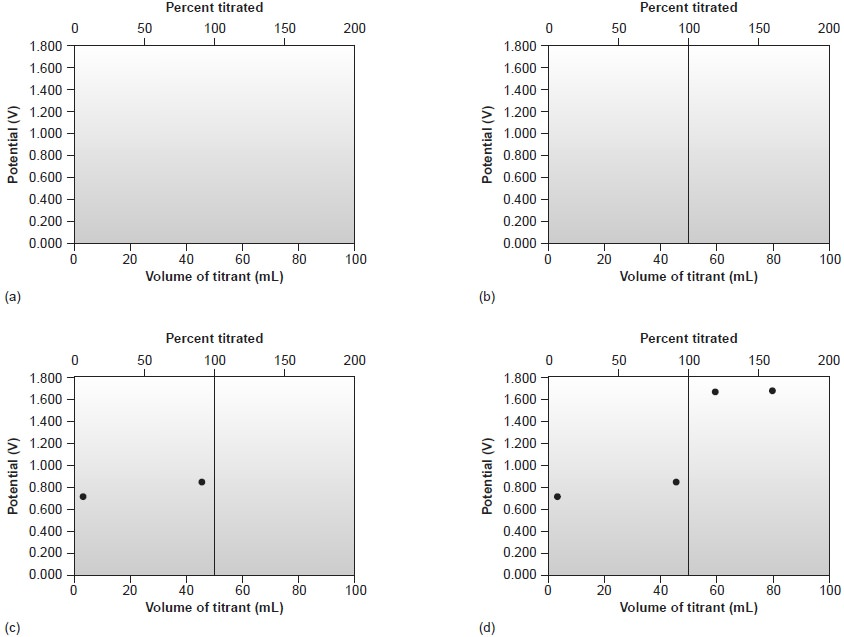Home | | Modern Analytical Chemistry | Redox Titration Curves

# Redox Titration CurvesTo evaluate a redox titration we must know the shape of its titration curve. In an acidâ€“base titration or a complexation titration, a titration curve shows the change in concentration of H3O+ (as pH) or Mn+ (as pM) as a function of the volume of titrant.

Redox Titration Curves

To evaluate a redox titration we must know the shape of its titration curve. In an acidâ€“base titration or a complexation titration, a titration curve shows the change in concentration of H3O+ (as pH) or Mn+ (as pM) as a function of the volume of titrant. For a redox titration, it is convenient to monitor electrochemical potential.

You will recall that the Nernst equation relates the electro- chemical potential to the concentrations of reactants and products participating in a redox reaction. Consider, for example, a titration in which the analyte in a reduced state, Ared, is titrated with a titrant in an oxidized state, Tox. The titration reaction is

Ared + Tox < == == >  Tred + Aox

The electrochemical potential for the reaction is the difference between the reduc- tion potentials for the reduction and oxidation half-reactions; thus,

Erxn = ETox/Tred â€“ EAox/Ared

After each addition of titrant, the reaction between the analyte and titrant reaches a state of equilibrium. The reactionâ€™s electrochemical potential, Erxn, therefore, is zero, and

ETox/Tred = EAox /Ared

Consequently, the potential for either half-reaction may be used to monitor the titrationâ€™s progress.

Before the equivalence point the titration mixture consists of appreciable quan- tities of both the oxidized and reduced forms of the analyte, but very little unreacted titrant. The potential, therefore, is best calculated using the Nernst equation for the analyteâ€™s half-reactionAlthough EËšAox /Ared is the standard-state potential for the analyteâ€™s half-reaction, a matrix-dependent formal potential is used in its place. After the equivalence point, the potential is easiest to calculate using the Nernst equation for the titrantâ€™s half-reaction, since significant quantities of its oxidized and reduced forms are present.## Calculating the Titration Curve

As an example, letâ€™s calculate the titration curve for the titration of 50.0 mL of 0.100 M Fe2+ with 0.100 M Ce4+ in a matrix of 1 M HClO4. The reaction in this case isThe equilibrium constant for this reaction is quite large (it is approximately 6 x 1015), so we may assume that the analyte and titrant react completely.

The first task is to calculate the volume of Ce4+ needed to reach the equivalence point. From the stoichiometry of the reaction we knowgives the equivalence point volume as 50.0 mL.

Before the equivalence point the concentration of unreacted Fe2+ and the con- centration of Fe3+ produced by reaction 9.16 are easy to calculate. For this reason we find the potential using the Nernst equation for the analyteâ€™s half-reactionAt the equivalence point, the moles of Fe2+ initially present and the moles of Ce4+ added are equal. Because the equilibrium constant for reaction 9.16 is large, the concentrations of Fe2+ and Ce4+ are exceedingly small and difficult to calculate without resorting to a complex equilibrium problem. Consequently, we cannot cal- culate the potential at the equivalence point, Eeq, using just the Nernst equation for the analyteâ€™s half-reaction or the titrantâ€™s half-reaction. We can, however, calculate Eeq by combining the two Nernst equations. To do so we recognize that the poten- tials for the two half-reactions are the same; thusAdding together these two Nernst equations leaves us withAt the equivalence point, the titration reactionâ€™s stoichiometry requires thatThe ratio in the log term of equation 9.18, therefore, equals one and the log term is zero. Equation 9.18 simplifies toAfter the equivalence point, the concentrations of Ce3+ and excess Ce4+ are easy to calculate. The potential, therefore, is best calculated using the Nernst equation for the titrantâ€™s half-reaction.Substituting these concentrations into equation 9.19 gives the potential asAdditional results for this titration curve are shown in Table 9.17 and Figure 9.34## Sketching a Redox Titration Curve

As we have done for acidâ€“base and complexo- metric titrations, we now show how to quickly sketch a redox titration curve using a minimum number of calculations.Study Material, Lecturing Notes, Assignment, Reference, Wiki description explanation, brief detail
Modern Analytical Chemistry: Titrimetric Methods of Analysis : Redox Titration Curves |